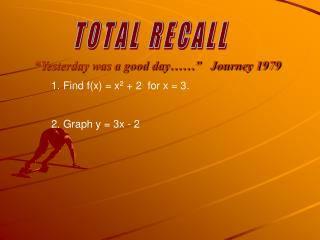# “Yesterday was a good day……” Journey 1979 - PowerPoint PPT PresentationDownload Presentation“Yesterday was a good day……” Journey 1979

“Yesterday was a good day……” Journey 1979Download Presentation## “Yesterday was a good day……” Journey 1979

- - - - - - - - - - - - - - - - - - - - - - - - - - - E N D - - - - - - - - - - - - - - - - - - - - - - - - - - -
##### Presentation Transcript

1. “Yesterday was a good day……” Journey 1979 T O T A L R E C A L L • Find f(x) = x2 + 2 for x = 3. • Graph y = 3x - 2

2. “Yesterday was a good day……” Journey 1979 T O T A L R E C A L L • Find f(x) = x2 + 2 for x = 3. • Answer: Replace 3 for ‘x’: • Graph y = 3x - 2

3. “Yesterday was a good day……” Journey 1979 T O T A L R E C A L L • Find f(x) = x2 + 2 for x = 3. • Answer: Replace 3 for ‘x’: • f(x) = 32 + 2 = 9 + 2 = 11 • Graph y = 3x - 2

4. “Yesterday was a good day……” Journey 1979 T O T A L R E C A L L • Find f(x) = x2 + 2 for x = 3. • Answer: Replace 3 for ‘x’: • f(x) = 32 + 2 = 9 + 2 = 11 • Graph y = 3x - 2

5. “Yesterday was a good day……” Journey 1979 T O T A L R E C A L L • Find f(x) = x2 + 2 for x = 3. • Answer: Replace 3 for ‘x’: • f(x) = 32 + 2 = 9 + 2 = 11 • Graph y = 3x - 2

6. “Yesterday was a good day……” Journey 1979 T O T A L R E C A L L • Find f(x) = x2 + 2 for x = 3. • Answer: Replace 3 for ‘x’: • f(x) = 32 + 2 = 9 + 2 = 11 • Graph y = 3x - 2

7. “Yesterday was a good day……” Journey 1979 T O T A L R E C A L L • Find f(x) = x2 + 2 for x = 3. • Answer: Replace 3 for ‘x’: • f(x) = 32 + 2 = 9 + 2 = 11 • Graph y = 3x - 2

8. “Yesterday was a good day……” Journey 1979 T O T A L R E C A L L • Find f(x) = x2 + 2 for x = 3. • Answer: Replace 3 for ‘x’: • f(x) = 32 + 2 = 9 + 2 = 11 • Graph y = 3x - 2